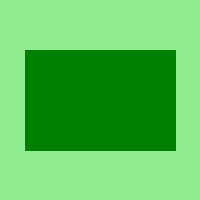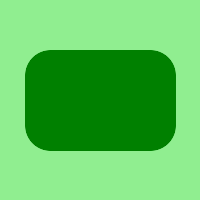Skip to content
Related Articles
Wand rectangle() function in Python
• Last Updated : 10 May, 2020

rectangle() function, as the name describes this function is used to draw a circle using wand.drawing object in Python. rectangle takes many arguments like left, top, right, bottom, width, height etc.

Syntax : wand.drawing.rectangle(left, top, right, bottom, width, height, radius, xradius, yradius)

Parameters :

ParameterInput TypeDescription
leftnumbers.Realx-offset of the rectangle to draw.
topnumbers.Realy-offset of the rectangle to draw.
rightnumbers.Realsecond x-offset of the rectangle to draw. this parameter and width parameter are exclusive each other.
bottomnumbers.Realsecond y-offset of the rectangle to draw. this parameter and height parameter are exclusive each other.
widthnumbers.Realthe width of the rectangle to draw. this parameter and right parameter are exclusive each other.
heightnumbers.Realthe height of the rectangle to draw. this parameter and bottom parameter are exclusive each other.
radiusnumbers.Realthe corner rounding. this is a short-cut for setting both xradius, and yradius.
xradiusnumbers.Realthe xradius corner in horizontal direction..
yradiusnumbers.Realthe yradius corner in vertical direction.

Example #1:

 `# Import different modules of wand``from` `wand.image ``import` `Image``from` `wand.drawing ``import` `Drawing``from` `wand.color ``import` `Color``import` `math``  ` `with Drawing() as draw:``    ``draw.fill_color ``=` `Color(``'GREEN'``)``    ``draw.rectangle(left ``=` `25``, top ``=` `50``, ``                   ``right ``=` `175``, bottom ``=` `150``)`` ` `    ``with Image(width ``=` `200``, height ``=` `200``, background ``=` `Color(``'lightgreen'``)) as image:``        ``draw(image)``        ``image.save(filename ``=` `"rectangle.png"``)`

Output:Example #2:
setting corner-radius for the rectangle.

 `# Import different modules of wand``from` `wand.image ``import` `Image``from` `wand.drawing ``import` `Drawing``from` `wand.color ``import` `Color``import` `math``  ` `with Drawing() as draw:``    ``draw.fill_color ``=` `Color(``'GREEN'``)``    ``draw.rectangle(left ``=` `25``, top ``=` `50``, right ``=` `175``,``                          ``bottom ``=` `150``, radius ``=` `25``)`` ` `    ``with Image(width ``=` `200``, height ``=` `200``, background ``=` `Color(``'lightgreen'``)) as image:``        ``draw(image)``        ``image.save(filename ``=` `"rectangle.png"``)`

Output:Attention geek! Strengthen your foundations with the Python Programming Foundation Course and learn the basics.

To begin with, your interview preparations Enhance your Data Structures concepts with the Python DS Course. And to begin with your Machine Learning Journey, join the Machine Learning – Basic Level Course

My Personal Notes arrow_drop_up